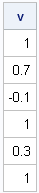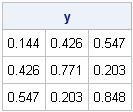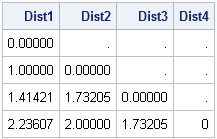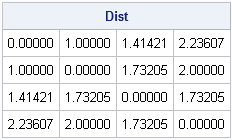Covariance, correlation, and distance matrices are a few examples of symmetric matrices that are frequently encountered in statistics. When you create a symmetric matrix, you only need to specify the lower triangular portion of the matrix. The VECH and SQRVECH functions, which were introduced in SAS/IML 9.3, are two functions that help you create and manipulate symmetric matrices.

### The VECH function: From lower triangular matrix to vector

The VECH function extracts the lower triangular elements of an NxN matrix. It stores these N(N+1)/2 elements in a vector. The VECH function, which is a standard function in matrix theory, gets its name because it forms the vector from half of the matrix: VECtor + Half = VECH.

The VECH function extracts the lower triangular elements in column-major order, as shown in the following example in which only the lower triangular elements are nonmissing:

```proc iml; corr = {1.0 . ., 0.7 1.0 ., -0.1 0.3 1.0}; v = vech(corr); print v;```### The SQRVECH function: From vector to square symmetric matrix

The SQRVECH function takes a vector with N(N+1)/2 elements and returns a symmetric NxN matrix. A clever application of the SQRVECH function is to generate a random symmetric matrix, as shown in the following example:

```/* Generate random symmetric matrix: 1. Generate N*(N+1)/2 elements for lower triangular 2. Use SQRVECH to form full symmetric matrix */ N=3; v = j(N*(N+1)/2, 1); /* allocate vector */ call randgen(v, "Uniform"); /* fill with random values */ y = sqrvech(v); /* create symmetric matrix */ print y[format=5.3];```### Reading a data matrix stored in lower triangular form

You can combine the VECH and SQRVECH functions to read in a matrix that is stored in a SAS data set in lower triangular form. For example, the following statements call PROC DISTANCE to compute the distance between four points in three-dimensional space. The distance matrix is stored in the distMatrix data set as a lower triangular matrix.

```data points; input x y z; datalines; 0 0 0 1 0 0 0 1 1 1 2 0 ;   proc distance data=points out=distMatrix; var interval(x y z); run; proc print noobs; run;```If you want to use this distance matrix in a SAS/IML program (or just want an easy way to convert it to a full symmetric matrix), you can extract the lower triangular elements by using the VECH function and then form the complete symmetric matrix by using the SQRVECH functions, as shown in the following statements:

```proc iml; use distMatrix; read all var _NUM_ into Dist; close distMatrix;   d = vech(Dist); /* extract lower triangular elements */ Dist = sqrvech(d); /* create dense symmetric matrix */ print Dist[format=7.5];```Share